# Metric SystemPage 1

#### WATCH ALL SLIDES

Slide 1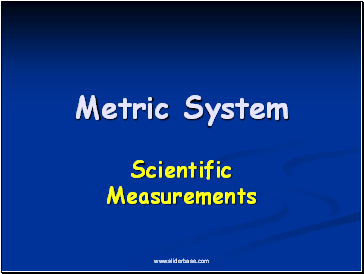Metric System

Scientific Measurements

Slide 2## Metric System

Developed by the French in the late 1700’s.

Based on powers of ten, so it is very easy to use.

Used by almost every country in the world, with the notable exception of the USA.

Especially used by scientists.

Abbreviated SI, which is French for Systeme International.

Slide 3## Metric Prefixes

Regardless of the unit, the entire metric system uses the same prefixes.

Common prefixes are:

kilo = 1000

centi = 1/100th

milli = 1/1000th

1 meter = 100 centimeters= 1000 millimeters

Slide 4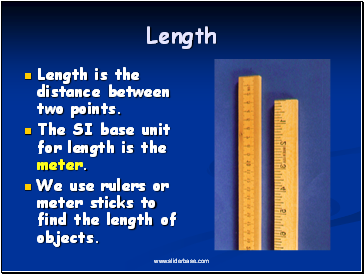## Length

Length is the distance between two points.

The SI base unit for length is the meter.

We use rulers or meter sticks to find the length of objects.

Slide 5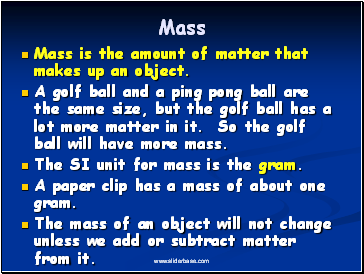## Mass

Mass is the amount of matter that makes up an object.

A golf ball and a ping pong ball are the same size, but the golf ball has a lot more matter in it. So the golf ball will have more mass.

The SI unit for mass is the gram.

A paper clip has a mass of about one gram.

The mass of an object will not change unless we add or subtract matter from it.

Slide 6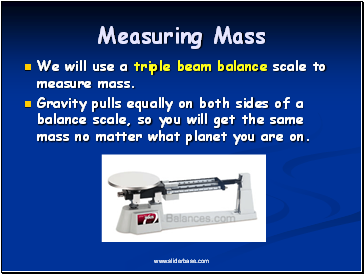## Measuring Mass

We will use a triple beam balance scale to measure mass.

Gravity pulls equally on both sides of a balance scale, so you will get the same mass no matter what planet you are on.

Slide 7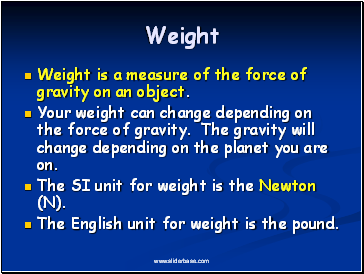## Weight

Weight is a measure of the force of gravity on an object.

Your weight can change depending on the force of gravity. The gravity will change depending on the planet you are on.

The SI unit for weight is the Newton (N).

The English unit for weight is the pound.

Slide 8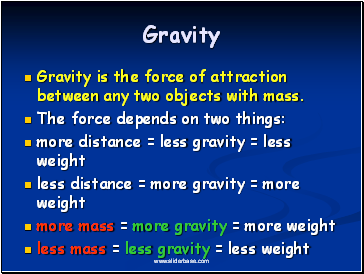## Gravity

Gravity is the force of attraction between any two objects with mass.

The force depends on two things:

more distance = less gravity = less weight

less distance = more gravity = more weight

more mass = more gravity = more weight

less mass = less gravity = less weight

Slide 9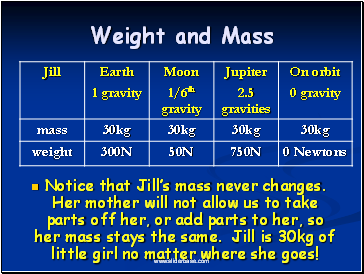## Weight and Mass

Notice that Jill’s mass never changes. Her mother will not allow us to take parts off her, or add parts to her, so her mass stays the same. Jill is 30kg of little girl no matter where she goes!

Go to page:
1  2  3  4